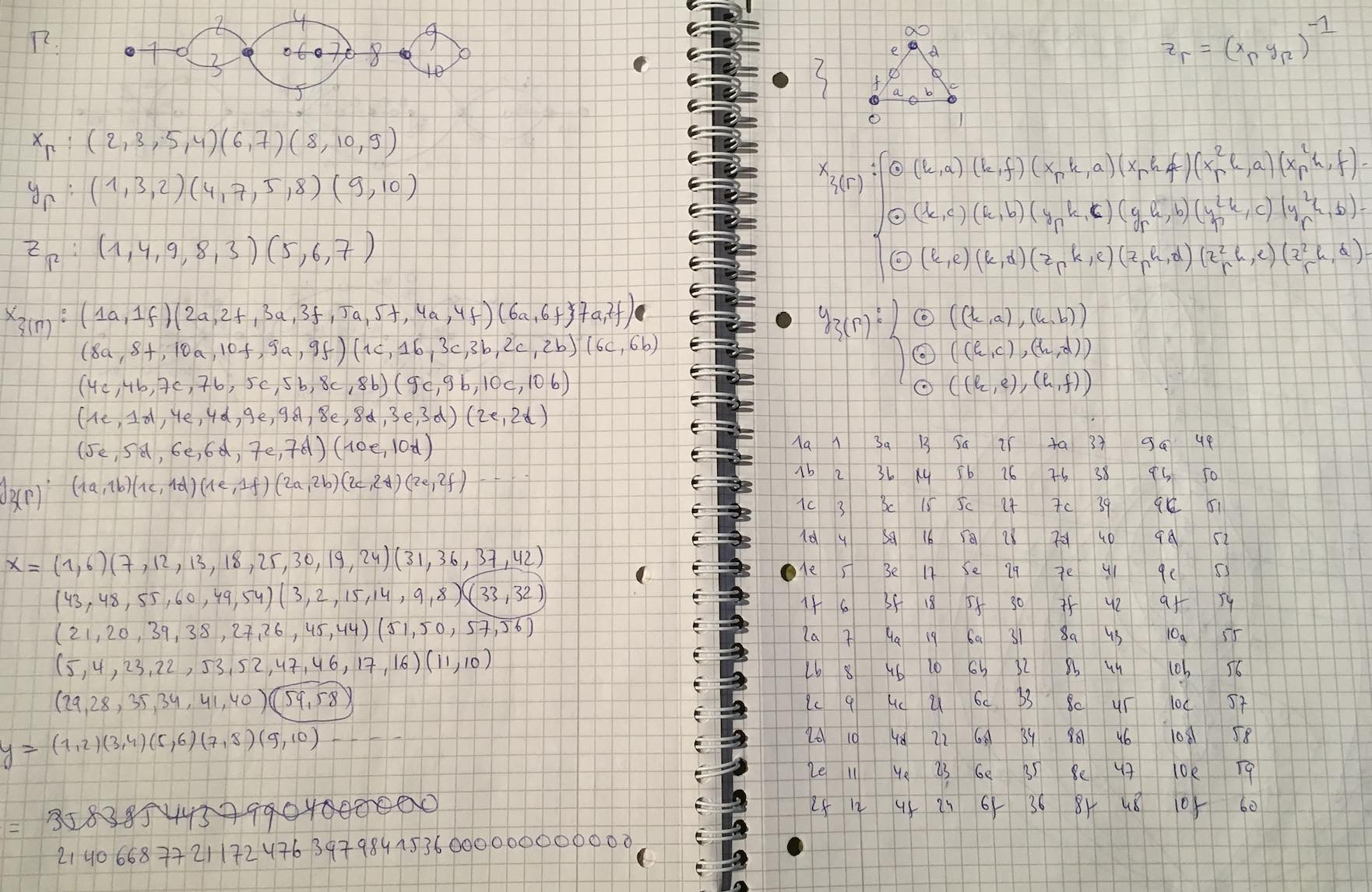## Complete chaos and Belyi-extenders

A Belyi-extender (or dessinflateur) is a rational function $q(t) = \frac{f(t)}{g(t)} \in \mathbb{Q}(t)$ that defines a map
$q : \mathbb{P}^1_{\mathbb{C}} \rightarrow \mathbb{P}^1_{\mathbb{C}}$
unramified outside $\{ 0,1,\infty \}$, and has the property that $q(\{ 0,1,\infty \}) \subseteq \{ 0,1,\infty \}$.

An example of such a Belyi-extender is the power map $q(t)=t^n$, which is totally ramified in $0$ and $\infty$ and we clearly have that $q(0)=0,~q(1)=1$ and $q(\infty)=\infty$.

The composition of two Belyi-extenders is again an extender, and we get a rather mysterious monoid $\mathcal{E}$ of all Belyi-extenders.

Very little seems to be known about this monoid. Its units form the symmetric group $S_3$ which is the automrphism group of $\mathbb{P}^1_{\mathbb{C}} – \{ 0,1,\infty \}$, and mapping an extender $q$ to its degree gives a monoid map $\mathcal{E} \rightarrow \mathbb{N}_+^{\times}$ to the multiplicative monoid of positive natural numbers.

If one relaxes the condition of $q(t) \in \mathbb{Q}(t)$ to being defined over its algebraic closure $\overline{\mathbb{Q}}$, then such maps/functions have been known for some time under the name of dynamical Belyi-functions, for example in Zvonkin’s Belyi Functions: Examples, Properties, and Applications (section 6).

Here, one is interested in the complex dynamical system of iterations of $q$, that is, the limit-behaviour of the orbits
$\{ z,q(z),q^2(z),q^3(z),… \}$
for all complex numbers $z \in \mathbb{C}$.

In general, the 2-sphere $\mathbb{P}^1_{\mathbb{C}} = S^2$ has a finite number of open sets (the Fatou domains) where the limit behaviour of the series is similar, and the union of these open sets is dense in $S^2$. The complement of the Fatou domains is the Julia set of the function, of which we might expect a nice fractal picture.

Let’s take again the power map $q(t)=t^n$. For a complex number $z$ lying outside the unit disc, the series $\{ z,z^n,z^{2n},… \}$ has limit point $\infty$ and for those lying inside the unit circle, this limit is $0$. So, here we have two Fatou domains (interior and exterior of the unit circle) and the Julia set of the power map is the (boring?) unit circle.

Fortunately, there are indeed dynamical Belyi-maps having a more pleasant looking Julia set, such as this one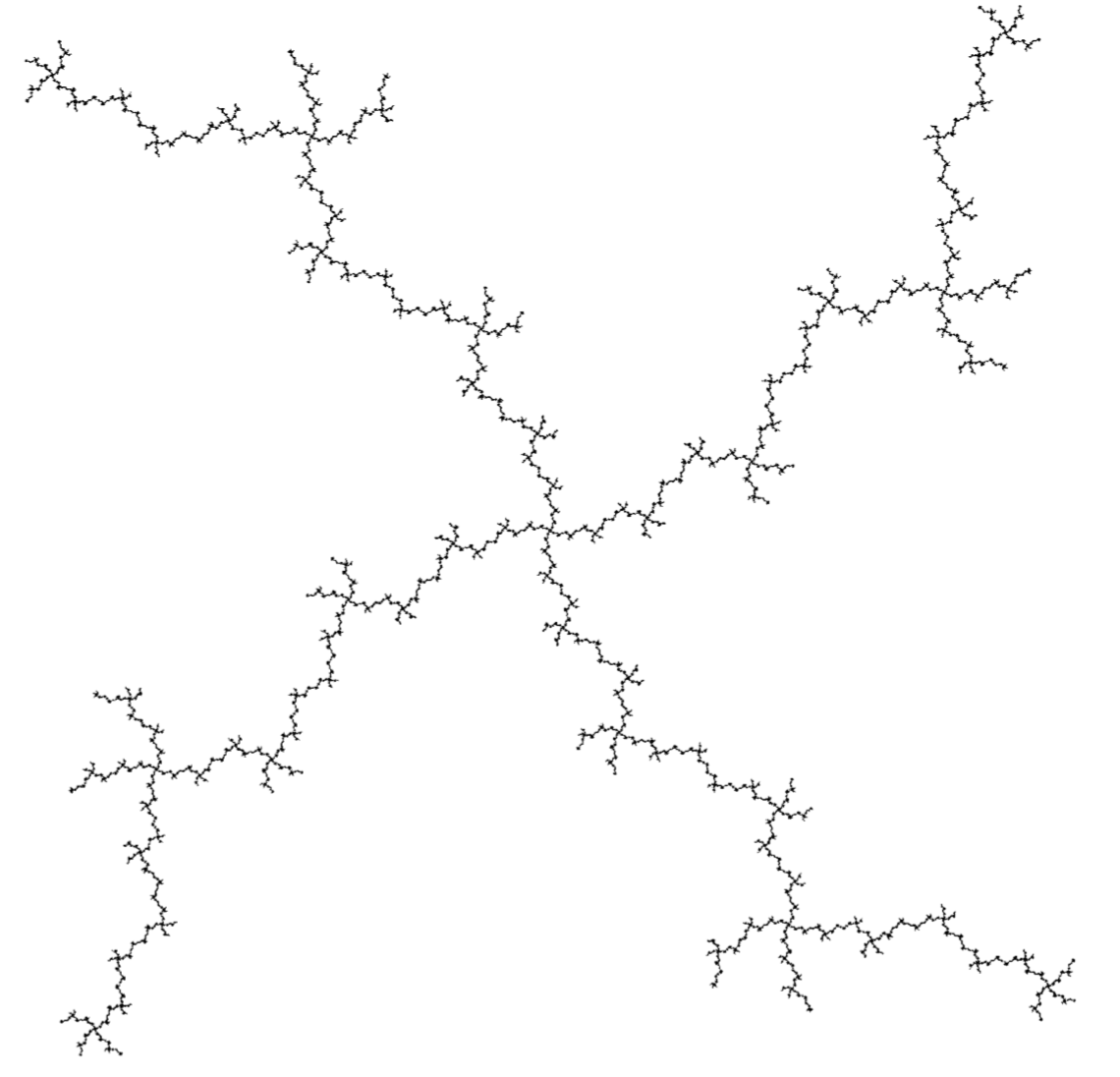But then, many dynamical Belyi-maps (and Belyi-extenders) are systems of an entirely different nature, they are completely chaotic, meaning that their Julia set is the whole $2$-sphere! Nowhere do we find an open region where points share the same limit behaviour… (the butterfly effect).

There’s a nice sufficient condition for chaotic behaviour, due to Dennis Sullivan, which is pretty easy to check for dynamical Belyi-maps.

A periodic point for $q(t)$ is a point $p \in S^2 = \mathbb{P}^1_{\mathbb{C}}$ such that $p = q^m(p)$ for some $m > 1$. A critical point is one such that either $q(p) = \infty$ or $q'(p)=0$.

Sullivan’s result is that $q(t)$ is completely chaotic when all its critical points $p$ become eventually periodic, that is some $q^k(p)$ is periodic, but $p$ itself is not periodic.

For a Belyi-map $q(t)$ the critical points are either comlex numbers mapping to $\infty$ or the inverse images of $0$ or $1$ (that is, the black or white dots in the dessin of $q(t)$) which are not leaf-vertices of the dessin.

Let’s do an example, already used by Sullivan himself:
$q(t) = (\frac{t-2}{t})^2$
This is a Belyi-function, and in fact a Belyi-extender as it is defined over $\mathbb{Q}$ and we have that $q(0)=\infty$, $q(1)=1$ and $q(\infty)=1$. The corresponding dessin is (inverse images of $\infty$ are marked with an $\ast$)The critical points $0$ and $2$ are not periodic, but they become eventually periodic:

$2 \rightarrow^q 0 \rightarrow^q \infty \rightarrow^q 1 \rightarrow^q 1$
and $1$ is periodic.

For a general Belyi-extender $q$, we have that the image under $q$ of any critical point is among $\{ 0,1,\infty \}$ and because we demand that $q(\{ 0,1,\infty \}) \subseteq \{ 0,1,\infty \}$, every critical point of $q$ eventually becomes periodic.

If we want to avoid the corresponding dynamical system to be completely chaotic, we have to ensure that one of the periodic points among $\{ 0,1,\infty \}$ (and there is at least one of those) must be critical.

Let’s consider the very special Belyi-extenders $q$ having the additional property that $q(0)=0$, $q(1)=1$ and $q(\infty)=\infty$, then all three of them are periodic.

So, the system is always completely chaotic unless the black dot at $0$ is not a leaf-vertex of the dessin, or the white dot at $1$ is not a leaf-vertex, or the degree of the region determined by the starred $\infty$ is at least two.

Going back to the mystery Manin-Marcolli sub-monoid of $\mathcal{E}$, it might explain why it is a good idea to restrict to very special Belyi-extenders having associated dessin a $2$-coloured tree, for then the periodic point $\infty$ is critical (the degree of the outside region is at least two), and therefore the conditions of Sullivan’s theorem are not satisfied. So, these Belyi-extenders do not necessarily have to be completely chaotic. (tbc)

## the mystery Manin-Marcolli monoid

A Belyi-extender (or dessinflateur) $\beta$ of degree $d$ is a quotient of two polynomials with rational coefficients
$\beta(t) = \frac{f(t)}{g(t)}$
with the special properties that for each complex number $c$ the polynomial equation of degree $d$ in $t$
$f(t)-c g(t)=0$
has $d$ distinct solutions, except perhaps for $c=0$ or $c=1$, and, in addition, we have that
$\beta(0),\beta(1),\beta(\infty) \in \{ 0,1,\infty \}$

Let’s take for instance the power maps $\beta_n(t)=t^n$.

For every $c$ the degree $n$ polynomial $t^n – c = 0$ has exactly $n$ distinct solutions, except for $c=0$, when there is just one. And, clearly we have that $0^n=0$, $1^n=1$ and $\infty^n=\infty$. So, $\beta_n$ is a Belyi-extender of degree $n$.

A cute observation being that if $\beta$ is a Belyi-extender of degree $d$, and $\beta’$ is an extender of degree $d’$, then $\beta \circ \beta’$ is again a Belyi-extender, this time of degree $d.d’$.

That is, Belyi-extenders form a monoid under composition!

In our example, $\beta_n \circ \beta_m = \beta_{n.m}$. So, the power-maps are a sub-monoid of the Belyi-extenders, isomorphic to the multiplicative monoid $\mathbb{N}_{\times}$ of strictly positive natural numbers.In their paper Quantum statistical mechanics of the absolute Galois group, Yuri I. Manin and Matilde Marcolli say they use the full monoid of Belyi-extenders to act on all Grothendieck’s dessins d’enfant.

But, they attach properties to these Belyi-extenders which they don’t have, in general. That’s fine, as they foresee in Remark 2.21 of their paper that the construction works equally well for any suitable sub-monoid, as long as this sub-monoid contains all power-map exenders.

I’m trying to figure out what the maximal mystery sub-monoid of extenders is satisfying all the properties they need for their proofs.

But first, let us see what Belyi-extenders have to do with dessins d’enfant.In his user-friendlier period, Grothendieck told us how to draw a picture, which he called a dessin d’enfant, of an extender $\beta(t) = \frac{f(t)}{g(t)}$ of degree $d$:

Look at all complex solutions of $f(t)=0$ and label them with a black dot (and add a black dot at $\infty$ if $\beta(\infty)=0$). Now, look at all complex solutions of $f(t)-g(t)=0$ and label them with a white dot (and add a white dot at $\infty$ if $\beta(\infty)=1$).

Now comes the fun part.

Because $\beta$ has exactly $d$ pre-images for all real numbers $\lambda$ in the open interval $(0,1)$ (and $\beta$ is continuous), we can connect the black dots with the white dots by $d$ edges (the pre-images of the open interval $(0,1)$), giving us a $2$-coloured graph.

For the power-maps $\beta_n(t)=t^n$, we have just one black dot at $0$ (being the only solution of $t^n=0$), and $n$ white dots at the $n$-th roots of unity (the solutions of $x^n-1=0$). Any $\lambda \in (0,1)$ has as its $n$ pre-images the numbers $\zeta_i.\sqrt[n]{\lambda}$ with $\zeta_i$ an $n$-th root of unity, so we get here as picture an $n$-star. Here for $n=5$: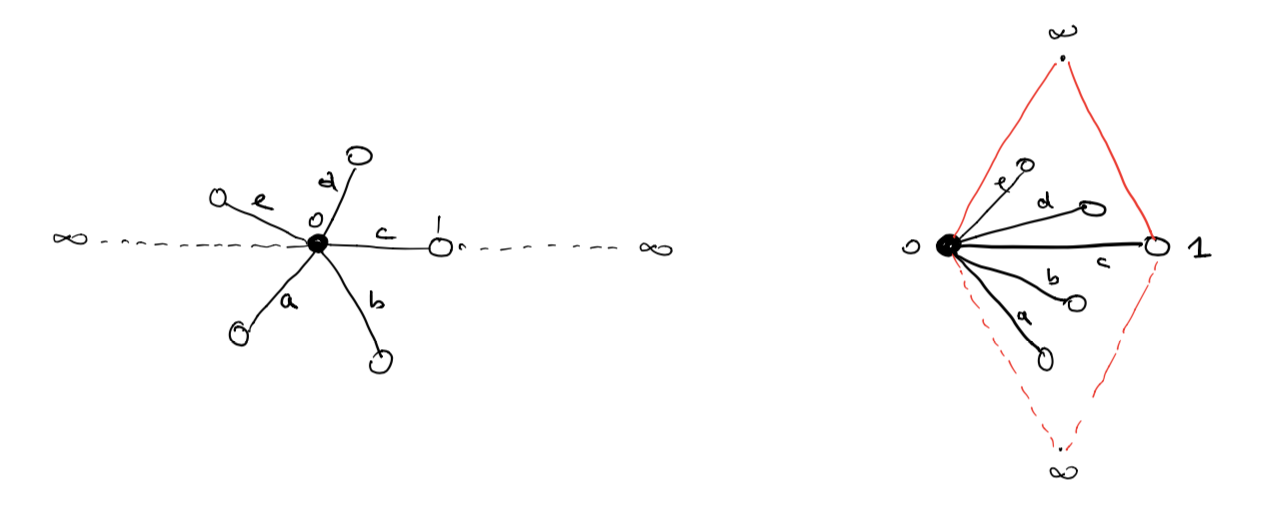This dessin should be viewed on the 2-sphere, with the antipodal point of $0$ being $\infty$, so projecting from $\infty$ gives a homeomorphism between the 2-sphere and $\mathbb{C} \cup \{ \infty \}$.

To get all information of the dessin (including possible dots at infinity) it is best to slice the sphere open along the real segments $(\infty,0)$ and $(1,\infty)$ and flatten it to form a ‘diamond’ with the upper triangle corresponding to the closed upper semisphere and the lower triangle to the open lower semisphere.

In the picture above, the right hand side is the dessin drawn in the diamond, and this representation will be important when we come to the action of extenders on more general Grothendieck dessins d’enfant.

Okay, let’s try to get some information about the monoid $\mathcal{E}$ of all Belyi-extenders.

What are its invertible elements?

Well, we’ve seen that the degree of a composition of two extenders is the product of their degrees, so invertible elements must have degree $1$, so are automorphisms of $\mathbb{P}^1_{\mathbb{C}} – \{ 0,1,\infty \} = S^2-\{ 0,1,\infty \}$ permuting the set $\{ 0,1,\infty \}$.

They form the symmetric group $S_3$ on $3$-letters and correspond to the Belyi-extenders
$t,~1-t,~\frac{1}{t},~\frac{1}{1-t},~\frac{t-1}{t},~\frac{t}{t-1}$
You can compose these units with an extender to get anther extender of the same degree where the roles of $0,1$ and $\infty$ are changed.

For example, if you want to colour all your white dots black and the black dots white, you compose with the unit $1-t$.

Manin and Marcolli use this and claim that you can transform any extender $\eta$ to an extender $\gamma$ by composing with a unit, such that $\gamma(0)=0, \gamma(1)=1$ and $\gamma(\infty)=\infty$.

That’s fine as long as your original extender $\eta$ maps $\{ 0,1,\infty \}$ onto $\{ 0,1,\infty \}$, but usually a Belyi-extender only maps into $\{ 0,1,\infty \}$.

Here are some extenders of degree three (taken from Melanie Wood’s paper Belyi-extending maps and the Galois action on dessins d’enfants):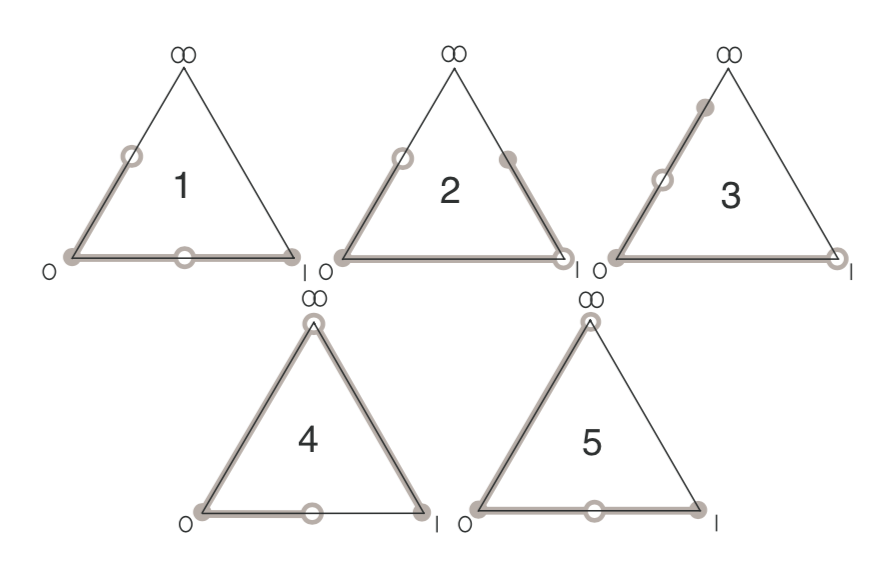with dessin $5$ corresponding to the Belyi-extender
$\beta(t) = \frac{t^2(t-1)}{(t-\frac{4}{3})^3}$
with $\beta(0)=0=\beta(1)$ and $\beta(\infty) = 1$.

So, a first property of the mystery Manin-Marcolli monoid $\mathcal{E}_{MMM}$ must surely be that all its elements $\gamma(t)$ map $\{ 0,1,\infty \}$ onto $\{ 0,1,\infty \}$, for they use this property a number of times, for instance to construct a monoid map
$\mathcal{E}_{MMM} \rightarrow M_2(\mathbb{Z})^+ \qquad \gamma \mapsto \begin{bmatrix} d & m-1 \\ 0 & 1 \end{bmatrix}$
where $d$ is the degree of $\gamma$ and $m$ is the number of black dots in the dessin (or white dots for that matter).

Further, they seem to believe that the dessin of any Belyi-extender must be a 2-coloured tree.

Already last time we’ve encountered a Belyi-extender $\zeta(t) = \frac{27 t^2(t-1)^2}{4(t^2-t+1)^3}$ with dessin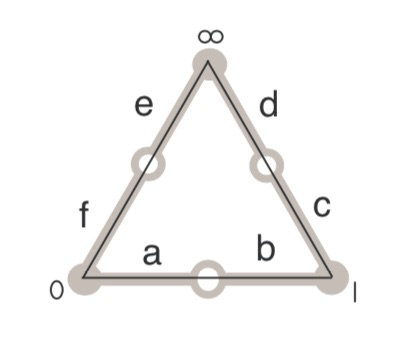But then, you may argue, this extender sends all of $0,1$ and $\infty$ to $0$, so it cannot belong to $\mathcal{E}_{MMM}$.

Here’s a trick to construct Belyi-extenders from Belyi-maps $\beta : \mathbb{P}^1 \rightarrow \mathbb{P}^1$, defined over $\mathbb{Q}$ and having the property that there are rational points in the fibers over $0,1$ and $\infty$.

Let’s take an example, the ‘monstrous dessin’ corresponding to the congruence subgroup $\Gamma_0(2)$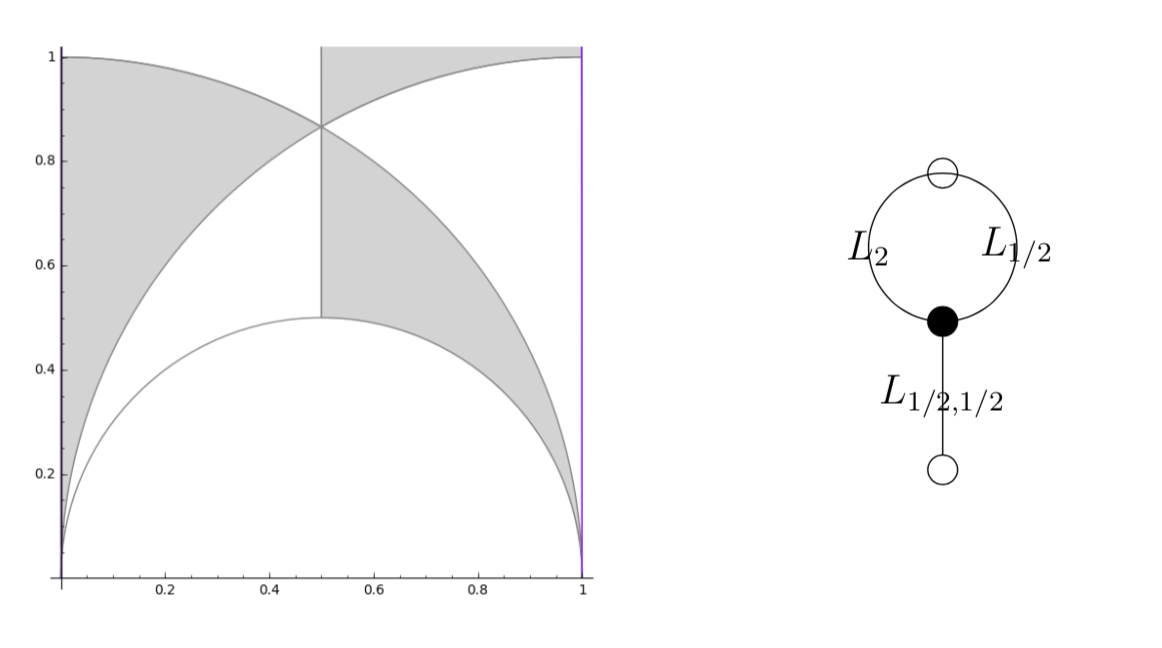with map $\beta(t) = \frac{(t+256)^3}{1728 t^2}$.

As it stands, $\beta$ is not a Belyi-extender because it does not map $1$ into $\{ 0,1,\infty \}$. But we have that
$-256 \in \beta^{-1}(0),~\infty \in \beta^{-1}(\infty),~\text{and}~512,-64 \in \beta^{-1}(1)$
(the last one follows from $(t+256)^2-1728 t^3=(t-512)^2(t+64)$).

We can now pre-compose $\beta$ with the automorphism (defined over $\mathbb{Q}$) sending $0$ to $-256$, $1$ to $-64$ and fixing $\infty$ to get a Belyi-extender
$\gamma(t) = \frac{(192t)^3}{1728(192t-256)^2}$
which maps $\gamma(0)=0,~\gamma(1)=1$ and $\gamma(\infty)=\infty$ (so belongs to $\mathcal{E}_{MMM}$) with the same dessin, which is not a tree,

That is, $\mathcal{E}_{MMM}$ can at best consist only of those Belyi-extenders $\gamma(t)$ that map $\{ 0,1,\infty \}$ onto $\{ 0,1,\infty \}$ and such that their dessin is a tree.

Let me stop, for now, by asking for a reference (or counterexample) to perhaps the most startling claim in the Manin-Marcolli paper, namely that any 2-coloured tree can be realised as the dessin of a Belyi-extender!

## Dessinflateurs

I’m trying to get into the latest Manin-Marcolli paper Quantum Statistical Mechanics of the Absolute Galois Group on how to create from Grothendieck’s dessins d’enfant a quantum system, generalising the Bost-Connes system to the non-Abelian part of the absolute Galois group $Gal(\overline{\mathbb{Q}}/\mathbb{Q})$.

In doing so they want to extend the action of the multiplicative monoid $\mathbb{N}_{\times}$ by power maps on the roots of unity to the action of a larger monoid on all dessins d’enfants.

Here they use an idea, originally due to Jordan Ellenberg, worked out by Melanie Wood in her paper Belyi-extending maps and the Galois action on dessins d’enfants.To grasp this, it’s best to remember what dessins have to do with Belyi maps, which are maps defined over $\overline{\mathbb{Q}}$
$\pi : \Sigma \rightarrow \mathbb{P}^1$
from a Riemann surface $\Sigma$ to the complex projective line (aka the 2-sphere), ramified only in $0,1$ and $\infty$. The dessin determining $\pi$ is the 2-coloured graph on the surface $\Sigma$ with as black vertices the pre-images of $0$, white vertices the pre-images of $1$ and these vertices are joined by the lifts of the closed interval $[0,1]$, so the number of edges is equal to the degree $d$ of the map.

Wood considers a very special subclass of these maps, which she calls Belyi-extender maps, of the form
$\gamma : \mathbb{P}^1 \rightarrow \mathbb{P}^1$
defined over $\mathbb{Q}$ with the additional property that $\gamma$ maps $\{ 0,1,\infty \}$ into $\{ 0,1,\infty \}$.

The upshot being that post-compositions of Belyi’s with Belyi-extenders $\gamma \circ \pi$ are again Belyi maps, and if two Belyi’s $\pi$ and $\pi’$ lie in the same Galois orbit, then so must all $\gamma \circ \pi$ and $\gamma \circ \pi’$.

The crucial Ellenberg-Wood idea is then to construct “new Galois invariants” of dessins by checking existing and easily computable Galois invariants on the dessins of the Belyi’s $\gamma \circ \pi$.

For this we need to know how to draw the dessin of $\gamma \circ \pi$ on $\Sigma$ if we know the dessins of $\pi$ and of the Belyi-extender $\gamma$. Here’s the procedure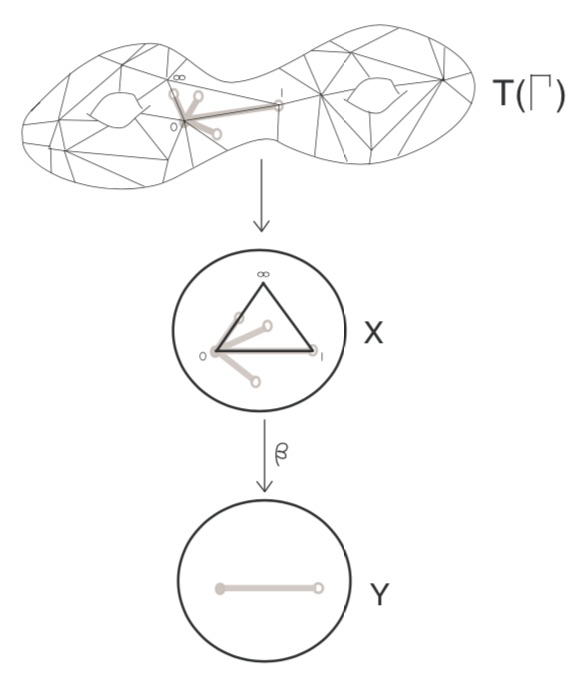Here, the middle dessin is that of the Belyi-extender $\gamma$ (which in this case is the power map $t \rightarrow t^4$) and the upper graph is the unmarked dessin of $\pi$.

One has to replace each of the black-white edges in the dessin of $\pi$ by the dessin of the expander $\gamma$, but one must be very careful in respecting the orientations on the two dessins. In the upper picture just one edge is replaced and one has to do this for all edges in a compatible manner.

Thus, a Belyi-expander $\gamma$ inflates the dessin $\pi$ with factor the degree of $\gamma$. For this reason i prefer to call them dessinflateurs, a contraction of dessin+inflator.

In her paper, Melanie Wood says she can separate dessins for which all known Galois invariants were the same, such as these two dessins,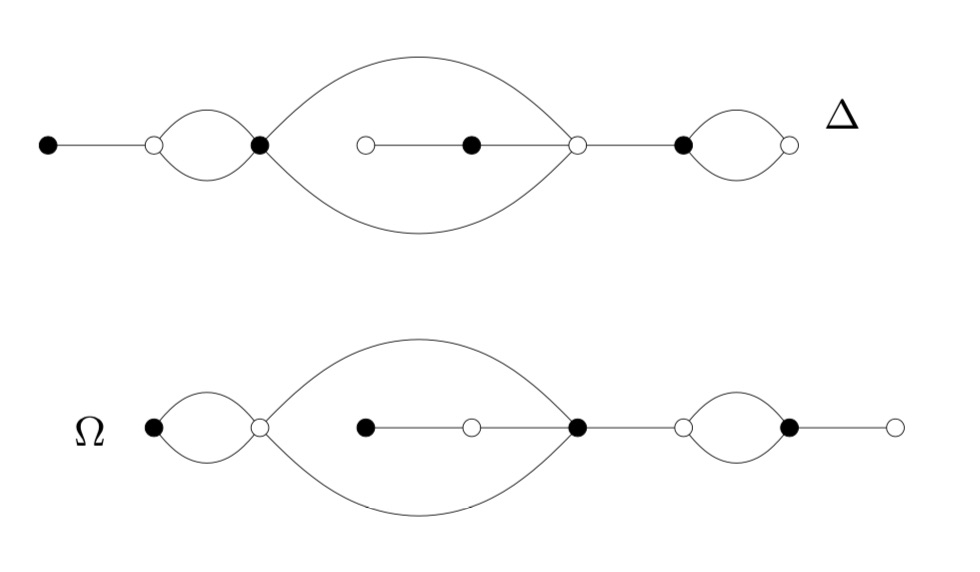by inflating them with a suitable Belyi-extender and computing the monodromy group of the inflated dessin.

This monodromy group is the permutation group generated by two elements, the first one gives the permutation on the edges given by walking counter-clockwise around all black vertices, the second by walking around all white vertices.

For example, by labelling the edges of $\Delta$, its monodromy is generated by the permutations $(2,3,5,4)(1,6)(8,10,9)$ and $(1,3,2)(4,7,5,8)(9,10)$ and GAP tells us that the order of this group is $1814400$. For $\Omega$ the generating permutations are $(1,2)(3,6,4,7)(8,9,10)$ and $(1,2,4,3)(5,6)(7,9,8)$, giving an isomorphic group.

Let’s inflate these dessins using the Belyi-extender $\gamma(t) = -\frac{27}{4}(t^3-t^2)$ with corresponding dessin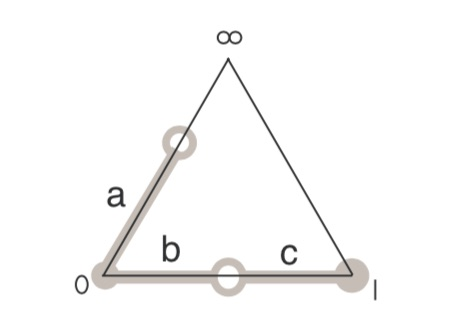It took me a couple of attempts before I got the inflated dessins correct (as i knew from Wood that this simple extender would not separate the dessins). Inflated $\Omega$ on top: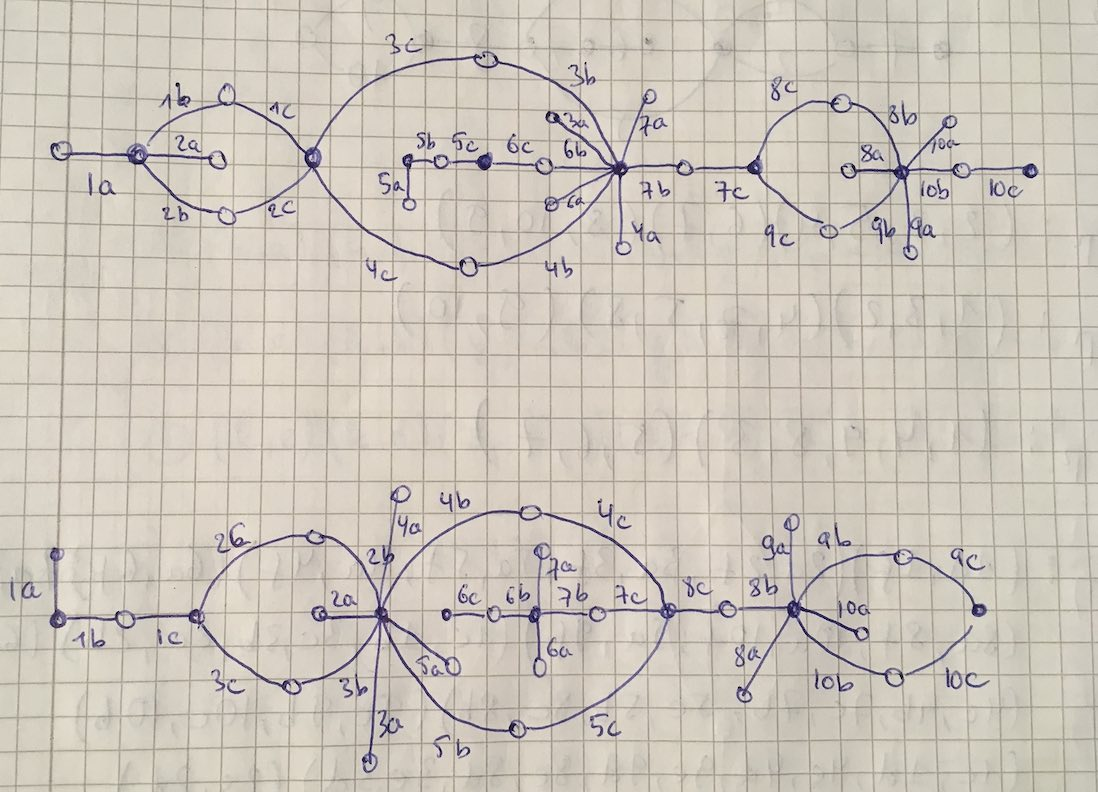Both dessins give a monodromy group of order $35838544379904000000$.

Now we’re ready to do serious work.

Melanie Wood uses in her paper the extender $\zeta(t)=\frac{27 t^2(t-1)^2}{4(t^2-t+1)^3}$ with associated dessinand says she can now separate the inflated dessins by the order of their monodromy groups. She gets for the inflated $\Delta$ the order $19752284160000$ and for inflated $\Omega$ the order $214066877211724763979841536000000000000$.

It’s very easy to make mistakes in these computations, so probably I did something horribly wrong but I get for both $\Delta$ and $\Omega$ that the order of the monodromy group of the inflated dessin is $214066877211724763979841536000000000000$.

I’d be very happy when someone would be able to spot the error!